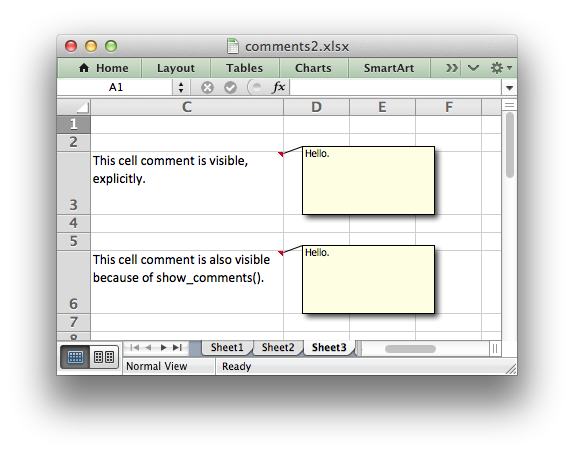Another example of adding cell comments to a worksheet. This example demonstrates most of the available comment formatting options. For more details see Working with Cell Comments.```###############################################################################
#
# An example of writing cell comments to a worksheet using Python and
# XlsxWriter.
#
# Each of the worksheets demonstrates different features of cell comments.
#
# Copyright 2013-2023, John McNamara, jmcnamara@cpan.org
#
import xlsxwriter

text_wrap = workbook.add_format({"text_wrap": 1, "valign": "top"})

###############################################################################
#
# Example 1. Demonstrates a simple cell comments without formatting.
#

# Set up some formatting.
worksheet1.set_column("C:C", 25)
worksheet1.set_row(2, 50)

# Simple ASCII string.
cell_text = "Hold the mouse over this cell to see the comment."

comment = "This is a comment."

worksheet1.write("C3", cell_text, text_wrap)
worksheet1.write_comment("C3", comment)

###############################################################################
#
# Example 2. Demonstrates visible and hidden comments.
#

# Set up some formatting.
worksheet2.set_column("C:C", 25)
worksheet2.set_row(2, 50)
worksheet2.set_row(5, 50)

cell_text = "This cell comment is visible."
comment = "Hello."

worksheet2.write("C3", cell_text, text_wrap)
worksheet2.write_comment("C3", comment, {"visible": True})

cell_text = "This cell comment isn't visible (the default)."

worksheet2.write("C6", cell_text, text_wrap)
worksheet2.write_comment("C6", comment)

###############################################################################
#
# Example 3. Demonstrates visible and hidden comments set at the worksheet
#            level.
#

# Set up some formatting.
worksheet3.set_column("C:C", 25)
worksheet3.set_row(2, 50)
worksheet3.set_row(5, 50)
worksheet3.set_row(8, 50)

# Make all comments on the worksheet visible.

cell_text = "This cell comment is visible, explicitly."
comment = "Hello."

worksheet3.write("C3", cell_text, text_wrap)
worksheet3.write_comment("C3", comment, {"visible": True})

cell_text = "This cell comment is also visible because of show_comments()."

worksheet3.write("C6", cell_text, text_wrap)
worksheet3.write_comment("C6", comment)

cell_text = "However, we can still override it locally."

worksheet3.write("C9", cell_text, text_wrap)
worksheet3.write_comment("C9", comment, {"visible": False})

###############################################################################
#
# Example 4. Demonstrates changes to the comment box dimensions.
#

# Set up some formatting.
worksheet4.set_column("C:C", 25)
worksheet4.set_row(2, 50)
worksheet4.set_row(5, 50)
worksheet4.set_row(8, 50)
worksheet4.set_row(15, 50)
worksheet4.set_row(18, 50)

cell_text = "This cell comment is default size."
comment = "Hello."

worksheet4.write("C3", cell_text, text_wrap)
worksheet4.write_comment("C3", comment)

cell_text = "This cell comment is twice as wide."

worksheet4.write("C6", cell_text, text_wrap)
worksheet4.write_comment("C6", comment, {"x_scale": 2})

cell_text = "This cell comment is twice as high."

worksheet4.write("C9", cell_text, text_wrap)
worksheet4.write_comment("C9", comment, {"y_scale": 2})

cell_text = "This cell comment is scaled in both directions."

worksheet4.write("C16", cell_text, text_wrap)
worksheet4.write_comment("C16", comment, {"x_scale": 1.2, "y_scale": 0.5})

cell_text = "This cell comment has width and height specified in pixels."

worksheet4.write("C19", cell_text, text_wrap)
worksheet4.write_comment("C19", comment, {"width": 200, "height": 50})

###############################################################################
#
# Example 5. Demonstrates changes to the cell comment position.
#
worksheet5.set_column("C:C", 25)
worksheet5.set_row(2, 50)
worksheet5.set_row(5, 50)
worksheet5.set_row(8, 50)
worksheet5.set_row(11, 50)

cell_text = "This cell comment is in the default position."
comment = "Hello."

worksheet5.write("C3", cell_text, text_wrap)
worksheet5.write_comment("C3", comment)

cell_text = "This cell comment has been moved to another cell."

worksheet5.write("C6", cell_text, text_wrap)
worksheet5.write_comment("C6", comment, {"start_cell": "E4"})

cell_text = "This cell comment has been moved to another cell."

worksheet5.write("C9", cell_text, text_wrap)
worksheet5.write_comment("C9", comment, {"start_row": 8, "start_col": 4})

cell_text = "This cell comment has been shifted within its default cell."

worksheet5.write("C12", cell_text, text_wrap)
worksheet5.write_comment("C12", comment, {"x_offset": 30, "y_offset": 12})

###############################################################################
#
# Example 6. Demonstrates changes to the comment background color.
#
worksheet6.set_column("C:C", 25)
worksheet6.set_row(2, 50)
worksheet6.set_row(5, 50)
worksheet6.set_row(8, 50)

cell_text = "This cell comment has a different color."
comment = "Hello."

worksheet6.write("C3", cell_text, text_wrap)
worksheet6.write_comment("C3", comment, {"color": "green"})

cell_text = "This cell comment has the default color."

worksheet6.write("C6", cell_text, text_wrap)
worksheet6.write_comment("C6", comment)

cell_text = "This cell comment has a different color."

worksheet6.write("C9", cell_text, text_wrap)
worksheet6.write_comment("C9", comment, {"color": "#CCFFCC"})

###############################################################################
#
# Example 7. Demonstrates how to set the cell comment author.
#
worksheet7.set_column("C:C", 30)
worksheet7.set_row(2, 50)
worksheet7.set_row(5, 50)

author = ""
cell = "C3"

cell_text = (
"Move the mouse over this cell and you will see 'Cell commented "
"by (blank)' in the status bar at the bottom"
)

comment = "Hello."

worksheet7.write(cell, cell_text, text_wrap)
worksheet7.write_comment(cell, comment)

author = "Python"
cell = "C6"
cell_text = (
"Move the mouse over this cell and you will see 'Cell commented "
"by Python' in the status bar at the bottom"
)

worksheet7.write(cell, cell_text, text_wrap)
worksheet7.write_comment(cell, comment, {"author": author})

###############################################################################
#
# Example 8. Demonstrates the need to explicitly set the row height.
#

# Set up some formatting.
worksheet8.set_column("C:C", 25)
worksheet8.set_row(2, 80)

cell_text = (
"The height of this row has been adjusted explicitly using "
"set_row(). The size of the comment box is adjusted "
"accordingly by XlsxWriter."
)

comment = "Hello."

worksheet8.write("C3", cell_text, text_wrap)
worksheet8.write_comment("C3", comment)

cell_text = (
"The height of this row has been adjusted by Excel due to the "
"text wrap property being set. Unfortunately this means that "
"the height of the row is unknown to XlsxWriter at run time "
"and thus the comment box is stretched as well.\n\n"
"Use set_row() to specify the row height explicitly to avoid "
"this problem."
)

worksheet8.write("C6", cell_text, text_wrap)
worksheet8.write_comment("C6", comment)

workbook.close()
```